# Tag: Klein

Here a list of saved pdf-files of previous NeverEndingBooks-posts on geometry in reverse chronological order.

Today we will explain how curves defined over
$\overline{\mathbb{Q}}$ determine permutation representations
of the carthographic groups. We have seen that any smooth projective
curve $C$ (a Riemann surface) defined over the algebraic
closure $\overline{\mathbb{Q}}$ of the rationals, defines a
_Belyi map_ $\xymatrix{C \ar[rr]^{\pi} & & \mathbb{P}^1}$ which is only ramified over the three points
$\\{ 0,1,\infty \\}$. By this we mean that there are
exactly $d$ points of $C$ lying over any other point
of $\mathbb{P}^1$ (we call $d$ the degree of
$\pi$) and that the number of points over $~0,1~$ and
$~\infty$ is smaller than $~d$. To such a map we
associate a _dessin d\’enfant_, a drawing on $C$ linking the
pre-images of $~0$ and $~1$ with exactly $d$
edges (the preimages of the open unit-interval). Next, we look at
the preimages of $~0$ and associate a permutation
$\tau_0$ of $~d$ letters to it by cycling
counter-clockwise around these preimages and recording the edges we
meet. We repeat this procedure for the preimages of $~1$ and
get another permutation $~\tau_1$. That is, we obtain a
subgroup of the symmetric group $\langle \tau_0,\tau_1 \rangle \subset S_d$ which is called the monodromy
group
of the covering $\pi$.For example, the
dessin on the right is
associated to a degree $8$ map $\mathbb{P}^1 \rightarrow \mathbb{P}^1$ and if we let the black (resp. starred) vertices be
the preimages of $~0$ (respectively of $~1$), then the
corresponding partitions are $\tau_0 = (2,3)(1,4,5,6)$
and $\tau_1 = (1,2,3)(5,7,8)$ and the monodromy group
is the alternating group $A_8$ (use
GAP ).

But wait! The map is also
ramified in $\infty$ so why don\’t we record also a
permutation $\tau_{\infty}$ and are able to compute it from
the dessin? (Note that all three partitions are needed if we want to
reconstruct $C$ from the $~d$ sheets as they encode in
which order the sheets fit together around the preimages). Well,
the monodromy group of a $\mathbb{P}^1$ covering ramified only
in three points is an epimorphic image of the fundamental
group
of the sphere
minus three points $\pi_1(\mathbb{P}^1 – { 0,1,\infty })$ That is, the group of all loops beginning and
ending in a basepoint upto homotopy (that is, two such loops are the
same if they can be transformed into each other in a continuous way
while avoiding the three points).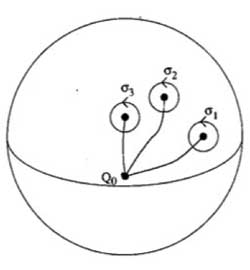This group is generated by loops
$\sigma_i$ running from the basepoint to nearby the i-th
point, doing a counter-clockwise walk around it and going back to be
basepoint $Q_0$ and the epimorphism to the monodromy group is given by sending

$\sigma_1 \mapsto \tau_0~\quad~\sigma_2 \mapsto \tau_1~\quad~\sigma_3 \mapsto \tau_{\infty}$

Now,
these three generators are not independent. In fact, this fundamental
group is

$\pi_1(\mathbb{P}^1 – \\{ 0,1,\infty \\}) = \langle \sigma_1,\sigma_2,\sigma_3~\mid~\sigma_1 \sigma_2 \sigma_3 = 1 \rangle$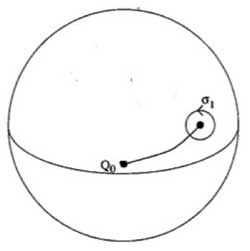To understand this, let us begin
with an easier case, that of the sphere minus one point. The fundamental group of the plane minus one point is
$~\mathbb{Z}$ as it encodes how many times we walk around the
point. However, on the sphere the situation is different as we can make
our walk around the point longer and longer until the whole walk is done
at the backside of the sphere and then we can just contract our walk to
the basepoint. So, there is just one type of walk on a sphere minus one
point (upto homotopy) whence this fundamental group is trivial. Next,
let us consider the sphere minus two points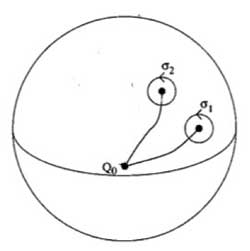Repeat the foregoing to the walk $\sigma_2$, that
is, strech the upper part of the circular tour all over the backside of
the sphere and then we see that we can move it to fit with the walk
$\sigma_1$ BUT for the orientation of the walk! That is, if we do this
modified walk $\sigma_1 \sigma_2^{\’}$ we just made the
trivial walk. So, this fundamental group is $\langle \sigma_1,\sigma_2~\mid~\sigma_1 \sigma_2 = 1 \rangle = \mathbb{Z}$ This is also the proof of the above claim. For,
we can modify the third walk $\sigma_3$ continuously so that
it becomes the walk $\sigma_1 \sigma_2$ but
with the reversed orientation ! As $\sigma_3 = (\sigma_1 \sigma_2)^{-1}$ this allows us to compute the
\’missing\’ permutation $\tau_{\infty} = (\tau_0 \tau_1)^{-1}$ In the example above, we obtain
$\tau_{\infty}= (1,2,6,5,8,7,4)(3)$ so it has two cycles
corresponding to the fact that the dessin has two regions (remember we
should draw ths on the sphere) : the head and the outer-region. Hence,
the pre-images of $\infty$ correspond to the different regions of the
dessin on the curve $C$. For another example,
consider the degree 168 map

$K \rightarrow \mathbb{P}^1$

which is the modified orbit map for the action of
$PSL_2(\mathbb{F}_7)$ on the Klein quartic.
The corresponding dessin is the heptagonal construction of the Klein
quartic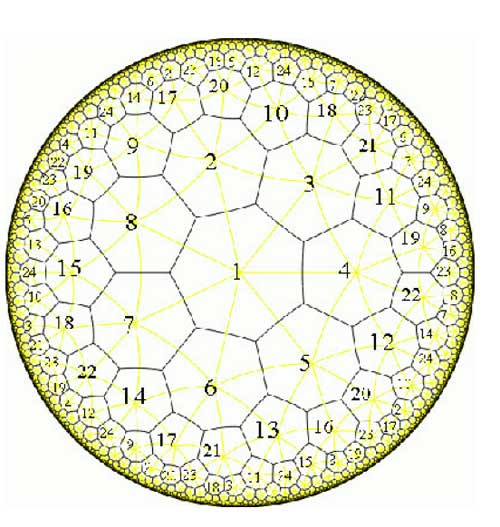Here, the pre-images of 1 correspond to the midpoints of the
84 edges of the polytope whereas the pre-images of 0 correspond to the
56 vertices. We can label the 168 half-edges by numbers such that
$\tau_0$ and $\tau_1$ are the standard generators b
resp. a of the 168-dimensional regular representation (see the atlas
page
).
Calculating with GAP the element $\tau_{\infty} = (\tau_0 \tau_1)^{-1} = (ba)^{-1}$ one finds that this permutation
consists of 24 cycles of length 7, so again, the pre-images of
$\infty$ lie one in each of the 24 heptagonal regions of the
Klein quartic. Now, we are in a position to relate curves defined
over $\overline{Q}$ via their Belyi-maps and corresponding
dessins to Grothendiecks carthographic groups $\Gamma(2)$,
$\Gamma_0(2)$ and $SL_2(\mathbb{Z})$. The
dessin gives a permutation representation of the monodromy group and
because the fundamental group of the sphere minus three
points $\pi_1(\mathbb{P}^1 – \\{ 0,1,\infty \\}) = \langle \sigma_1,\sigma_2,\sigma_3~\mid~\sigma_1 \sigma_2 \sigma_3 = 1 \rangle = \langle \sigma_1,\sigma_2 \rangle$ is the free group op two generators, we see that
any dessin determines a permutation representation of the congruence
subgroup $\Gamma(2)$ (see this
post
where we proved that this
group is free). A clean dessin is one for which one type of
vertex has all its valancies (the number of edges in the dessin meeting
the vertex) equal to one or two. (for example, the pre-images of 1 in
the Klein quartic-dessin or the pre-images of 1 in the monsieur Mathieu
example
) The corresponding
permutation $\tau_1$ then consists of 2-cycles and hence the
monodromy group gives a permutation representation of the free
product $C_{\infty} \ast C_2 = \Gamma_0(2)$ Finally, a clean dessin is said to be a
quilt dessin if also the other type of vertex has all its valancies
equal to one or three (as in the Klein quartic or Mathieu examples).
Then, the corresponding permutation has order 3 and for these
quilt-dessins the monodromy group gives a permutation representation of
the free product $C_2 \ast C_3 = PSL_2(\mathbb{Z})$ Next time we will see how this lead
Grothendieck to his anabelian geometric approach to the absolute Galois
group.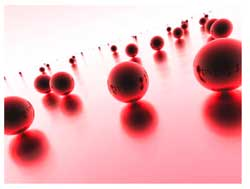Last time we have
seen that the noncommutative manifold of a Riemann surface can be viewed
as that Riemann surface together with a loop in each point. The extra
loop-structure tells us that all finite dimensional representations of
the coordinate ring can be found by separating over points and those
living at just one point are classified by the isoclasses of nilpotent
matrices, that is are parametrized by the partitions (corresponding
to the sizes of the Jordan blocks). In addition, these loops tell us
that the Riemann surface locally looks like a Riemann sphere, so an
equivalent mental picture of the local structure of this
noncommutative manifold is given by the picture on teh left, where the surface is part of the Riemann surface
and a sphere is placed at every point. Today we will consider
genuine noncommutative curves and describe their corresponding
noncommutative manifolds.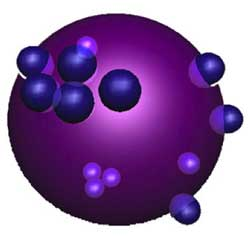Here, a mental picture of such a
_noncommutative sphere_ to keep in mind would be something
like the picture on the right. That is, in most points of the sphere we place as before again
a Riemann sphere but in a finite number of points a different phenomen
occurs : we get a cluster of infinitesimally nearby points. We
will explain this picture with an easy example. Consider the
complex plane $\mathbb{C}$, the points of which are just the
one-dimensional representations of the polynomial algebra in one
variable $\mathbb{C}[z]$ (any algebra map $\mathbb{C}[z] \rightarrow \mathbb{C}$ is fully determined by the image of z). On this plane we
have an automorphism of order two sending a complex number z to its
negative -z (so this automorphism can be seen as a point-reflexion
with center the zero element 0). This automorphism extends to
the polynomial algebra, again induced by sending z to -z. That
is, the image of a polynomial $f(z) \in \mathbb{C}[z]$ under this
automorphism is f(-z).

With this data we can form a noncommutative
algebra, the _skew-group algebra_ $\mathbb{C}[z] \ast C_2$ the
elements of which are either of the form $f(z) \ast e$ or $g(z) \ast g$ where
$C_2 = \langle g : g^2=e \rangle$ is the cyclic group of order two
generated by the automorphism g and f(z),g(z) are arbitrary
polynomials in z.

The multiplication on this algebra is determined by
the following rules

$(g(z) \ast g)(f(z) \ast e) = g(z)f(-z) \astg$ whereas $(f(z) \ast e)(g(z) \ast g) = f(z)g(z) \ast g$

$(f(z) \ast e)(g(z) \ast e) = f(z)g(z) \ast e$ whereas $(f(z) \ast g)(g(z)\ast g) = f(z)g(-z) \ast e$

That is, multiplication in the
$\mathbb{C}[z]$ factor is the usual multiplication, multiplication in
the $C_2$ factor is the usual group-multiplication but when we want
to get a polynomial from right to left over a group-element we have to
apply the corresponding automorphism to the polynomial (thats why we
call it a _skew_ group-algebra).

Alternatively, remark that as
a $\mathbb{C}$-algebra the skew-group algebra $\mathbb{C}[z] \ast C_2$ is
an algebra with unit element 1 = 1\aste and is generated by
the elements $X = z \ast e$ and $Y = 1 \ast g$ and that the defining
relations of the multiplication are

$Y^2 = 1$ and $Y.X =-X.Y$

hence another description would
be

$\mathbb{C}[z] \ast C_2 = \frac{\mathbb{C} \langle X,Y \rangle}{ (Y^2-1,XY+YX) }$

It can be shown that skew-group
algebras over the coordinate ring of smooth curves are _noncommutative
smooth algebras_ whence there is a noncommutative manifold associated
to them. Recall from last time the noncommutative manifold of a
smooth algebra A is a device to classify all finite dimensional
representations of A upto isomorphism
Let us therefore try to
determine some of these representations, starting with the
one-dimensional ones, that is, algebra maps from

$\mathbb{C}[z] \ast C_2 = \frac{\mathbb{C} \langle X,Y \rangle}{ (Y^2-1,XY+YX) } \rightarrow \mathbb{C}$

Such a map is determined by the image of X and that of
Y. Now, as $Y^2=1$ we have just two choices for the image of Y
namely +1 or -1. But then, as the image is a commutative algebra
and as XY+YX=0 we must have that the image of 2XY is zero whence the
image of X must be zero. That is, we have only
two
one-dimensional representations, namely $S_+ : X \rightarrow 0, Y \rightarrow 1$
and $S_- : X \rightarrow 0, Y \rightarrow -1$

This is odd! Can
it be that our noncommutative manifold has just 2 points? Of course not.
In fact, these two points are the exceptional ones giving us a cluster
of nearby points (see below) whereas most points of our
noncommutative manifold will correspond to 2-dimensional
representations!

So, let’s hunt them down. The
center of $\mathbb{C}[z]\ast C_2$ (that is, the elements commuting with
all others) consists of all elements of the form $f(z)\ast e$ with f an
_even_ polynomial, that is, f(z)=f(-z) (because it has to commute
with 1\ast g), so is equal to the subalgebra $\mathbb{C}[z^2]\ast e$.

The
manifold corresponding to this subring is again the complex plane
$\mathbb{C}$ of which the points correspond to all one-dimensional
representations of $\mathbb{C}[z^2]\ast e$ (determined by the image of
$z^2\ast e$).

We will now show that to each point of $\mathbb{C} – { 0 }$
corresponds a simple 2-dimensional representation of
$\mathbb{C}[z]\ast C_2$.

If a is not zero, we will consider the
quotient of the skew-group algebra modulo the twosided ideal generated
by $z^2\ast e-a$. It turns out
that

$\frac{\mathbb{C}[z]\ast C_2}{(z^2\aste-a)} = \frac{\mathbb{C}[z]}{(z^2-a)} \ast C_2 = (\frac{\mathbb{C}[z]}{(z-\sqrt{a})} \oplus \frac{\mathbb{C}[z]}{(z+\sqrt{a})}) \ast C_2 = (\mathbb{C} \oplus \mathbb{C}) \ast C_2$

where the skew-group algebra on the
right is given by the automorphism g on $\mathbb{C} \oplus \mathbb{C}$ interchanging the two factors. If you want to
become more familiar with working in skew-group algebras work out the
details of the fact that there is an algebra-isomorphism between
$(\mathbb{C} \oplus \mathbb{C}) \ast C_2$ and the algebra of $2 \times 2$ matrices $M_2(\mathbb{C})$. Here is the
identification

$~(1,0)\aste \rightarrow \begin{bmatrix} 1 & 0 \\ 0 & 0 \end{bmatrix}$

$~(0,1)\aste \rightarrow \begin{bmatrix} 0 & 0 \\ 0 & 1 \end{bmatrix}$

$~(1,0)\astg \rightarrow \begin{bmatrix} 0 & 1 \\ 0 & 0 \end{bmatrix}$

$~(0,1)\astg \rightarrow \begin{bmatrix} 0 & 0 \\ 1 & 0 \end{bmatrix}$

so you have to verify that multiplication
on the left hand side (that is in $(\mathbb{C} \oplus \mathbb{C}) \ast C_2$) coincides with matrix-multiplication of the associated
matrices.

Okay, this begins to look like what we are after. To
every point of the complex plane minus zero (or to every point of the
Riemann sphere minus the two points ${ 0,\infty }$) we have
associated a two-dimensional simple representation of the skew-group
algebra (btw. simple means that the matrices determined by the images
of X and Y generate the whole matrix-algebra).

In fact, we
now have already classified ‘most’ of the finite dimensional
representations of $\mathbb{C}[z]\ast C_2$, namely those n-dimensional
representations

$\mathbb{C}[z]\ast C_2 = \frac{\mathbb{C} \langle X,Y \rangle}{(Y^2-1,XY+YX)} \rightarrow M_n(\mathbb{C})$

for which the image of X is an invertible $n \times n$ matrix. We can show that such representations only exist when
n is an even number, say n=2m and that any such representation is
again determined by the geometric/combinatorial data we found last time
for a Riemann surface.

That is, It is determined by a finite
number ${ P_1,\dots,P_k }$ of points from $\mathbb{C} – 0$ where
k is at most m. For each index i we have a positive
number $a_i$ such that $a_1+\dots+a_k=m$ and finally for each i we
also have a partition of $a_i$.

That is our noncommutative
manifold looks like all points of $\mathbb{C}-0$ with one loop in each
point. However, we have to remember that each point now determines a
simple 2-dimensional representation and that in order to get all
finite dimensional representations with det(X) non-zero we have to
scale up representations of $\mathbb{C}[z^2]$ by a factor two.
The technical term here is that of a Morita equivalence (or that the
noncommutative algebra is an Azumaya algebra over
$\mathbb{C}-0$).

What about the remaining representations, that
is, those for which Det(X)=0? We have already seen that there are two
1-dimensional representations $S_+$ and $S_-$ lying over 0, so how
do they fit in our noncommutative manifold? Should we consider them as
two points and draw also a loop in each of them or do we have to do
something different? Rememer that drawing a loop means in our
geometry -> representation dictionary that the representations
living at that point are classified in the same way as nilpotent
matrices.

Hence, drawing a loop in $S_+$ would mean that we have a
2-dimensional representation of $\mathbb{C}[z]\ast C_2$ (different from
$S_+ \oplus S_+$) and any such representation must correspond to
matrices

$X \rightarrow \begin{bmatrix} 0 & 1 \\ 0 & 0 \end{bmatrix}$ and $Y \rightarrow \begin{bmatrix} 1 & 0 \\ 0 & 1 \end{bmatrix}$

But this is not possible as these matrices do
_not_ satisfy the relation XY+YX=0. Hence, there is no loop in $S_+$
and similarly also no loop in $S_-$.

However, there are non
semi-simple two dimensional representations build out of the simples
$S_+$ and $S_-$. For, consider the matrices

$X \rightarrow \begin{bmatrix} 0 & 1 \\ 0 & 0 \end{bmatrix}$ and $Y \rightarrow \begin{bmatrix} 1 & 0 \\ 0 & -1 \end{bmatrix}$

then these
matrices _do_ satisfy XY+YX=0! (and there is another matrix-pair
interchanging $\pm 1$ in the Y-matrix). In erudite terminology this
says that there is a _nontrivial extension_ between $S_+$ and $S_-$
and one between $S_-$ and $S_+$.

In our dictionary we will encode this
information by the picture

$\xymatrix{\vtx{} \ar@/^2ex/[rr] & & \vtx{} \ar@/^2ex/[ll]}$

where the two
vertices correspond to the points $S_+$ and $S_-$ and the arrows
represent the observed extensions. In fact, this data suffices to finish
our classification project of finite dimensional representations of
the noncommutative curve $\mathbb{C}[z] \ast C_2$.

Those with Det(X)=0
are of the form : $R \oplus T$ where R is a representation with
invertible X-matrix (which we classified before) and T is a direct
sum of representations involving only the simple factors $S_+$ and
$S_-$ and obtained by iterating the 2-dimensional idea. That is, for
each factor the Y-matrix has alternating $\pm 1$ along the diagonal
and the X-matrix is the full nilpotent Jordan-matrix.

So
here is our picture of the noncommutative manifold of the
noncommutative curve $\mathbb{C}[z]\ast C_2$
: the points are all points
of $\mathbb{C}-0$ together with one loop in each of them together
with two points lying over 0 where we draw the above picture of arrows
between them. One should view these two points as lying
infinetesimally close to each other and the gluing
data

$\xymatrix{\vtx{} \ar@/^2ex/[rr] & & \vtx{} \ar@/^2ex/[ll]}$

contains enough information to determine
that all other points of the noncommutative manifold in the vicinity of
this cluster should be two dimensional simples! The methods used
in this simple minded example are strong enough to determine the
structure of the noncommutative manifold of _any_ noncommutative curve.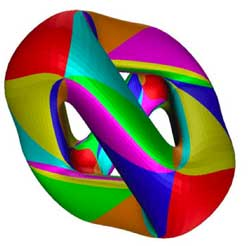So, let us look at a real-life example. Once again, take the
Kleinian quartic In a previous
course-post we recalled that
there is an action by automorphisms on the Klein quartic K by the
finite simple group $PSL_2(\mathbb{F}_7)$ of order 168. Hence, we
can form the noncommutative Klein-quartic $K \ast PSL_2(\mathbb{F}_7)$
(take affine pieces consisting of complements of orbits and do the
skew-group algebra construction on them and then glue these pieces
together again).

We have also seen that the orbits are classified
under a Belyi-map $K \rightarrow \mathbb{P}^1_{\mathbb{C}}$ and that this map
had the property that over any point of $\mathbb{P}^1_{\mathbb{C}} – { 0,1,\infty }$ there is an orbit consisting of 168 points
whereas over 0 (resp. 1 and $\infty$) there is an orbit
consisting of 56 (resp. 84 and 24 points).

So what is
the noncommutative manifold associated to the noncommutative Kleinian?
Well, it looks like the picture we had at the start of this
postFor all but three points of the Riemann sphere
$\mathbb{P}^1 – { 0,1,\infty }$ we have one point and one loop
(corresponding to a simple 168-dimensional representation of $K \ast PSL_2(\mathbb{F}_7)$) together with clusters of infinitesimally nearby
points lying over 0,1 and $\infty$ (the cluster over 0
is depicted, the two others only indicated).

Over 0 we have
three points connected by the diagram

$\xymatrix{& \vtx{} \ar[ddl] & \\ & & \\ \vtx{} \ar[rr] & & \vtx{} \ar[uul]}$

where each of the vertices corresponds to a
simple 56-dimensional representation. Over 1 we have a cluster of
two points corresponding to 84-dimensional simples and connected by
the picture we had in the $\mathbb{C}[z]\ast C_2$ example).

Finally,
over $\infty$ we have the most interesting cluster, consisting of the
seven dwarfs (each corresponding to a simple representation of dimension
24) and connected to each other via the
picture

$\xymatrix{& & \vtx{} \ar[dll] & & \\ \vtx{} \ar[d] & & & & \vtx{} \ar[ull] \\ \vtx{} \ar[dr] & & & & \vtx{} \ar[u] \\ & \vtx{} \ar[rr] & & \vtx{} \ar[ur] &}$

Again, this noncommutative manifold gives us
all information needed to give a complete classification of all finite
dimensional $K \ast PSL_2(\mathbb{F}_7)$-representations. One
can prove that all exceptional clusters of points for a noncommutative
curve are connected by a cyclic quiver as the ones above. However, these
examples are still pretty tame (in more than one sense) as these
noncommutative algebras are finite over their centers, are Noetherian
etc. The situation will become a lot wilder when we come to exotic
situations such as the noncommutative manifold of
$SL_2(\mathbb{Z})$…

The
natural habitat of this lesson is a bit further down the course, but it
was called into existence by a comment/question by
Kea

I don’t yet quite see where the nc
manifolds are, but I guess that’s coming.

As
I’m enjoying telling about all sorts of sources of finite dimensional
representations of $SL_2(\mathbb{Z})$ (and will carry on doing so for
some time), more people may begin to wonder where I’m heading. For this
reason I’ll do a couple of very elementary posts on simple examples of
noncommutative manifolds.

I realize it is ‘bon ton’ these days
to say that noncommutative manifolds are virtual objects associated to
noncommutative algebras and that the calculation of certain invariants
of these algebras gives insight into the topology and/or geometry of
these non-existent spaces. My own attitude to noncommutative geometry is
different : to me, noncommutative manifolds are genuine sets of points
equipped with a topology and other structures which I can use as a
mnemotechnic device to solve the problem of interest to me which is the
classification of all finite dimensional representations of a smooth
noncommutative algebra.

Hence, when I speak of the
‘noncommutative manifold of $SL_2(\mathbb{Z})$’ Im after an object
containing enough information to allow me (at least in principle) to
classify the isomorphism classes of all finite dimensional
$SL_2(\mathbb{Z})$-representations. The whole point of this course is
to show that such an object exists and that we can make explicit
an elementary question :

Riemann surfaces are examples of
noncommutative manifolds, so what is the noncommutative picture of
them?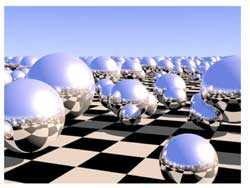I’ve browsed the Google-pictures a bit and a picture
coming close to my mental image of the noncommutative manifold of a
Riemann surface locally looks like the picture on the left. Here, the checkerboard-surface is part of the Riemann surface
and the extra structure consists in putting in each point of the Riemann
surface a sphere, reflecting the local structure of the Riemann surface
near the point. In fact, my picture is slightly different : I want to
draw a loop in each point of the Riemann surface, but Ill explain why
the two pictures are equivalent and why they present a solution to the
problem of classifying all finite dimensional representations of the
Riemann surface. After all why do we draw and study Riemann
surfaces? Because we are interested in the solutions to equations. For
example, the points of the _Kleinian quartic Riemann
surface_give us all solutions tex \in
\mathbb{C}^3 $to the equation$X^3Y+Y^3Z+Z^3X=0 $. If (a,b,c) is such a solution, then so are all scalar multiples$(\lambda a,\lambda
b,\lambda c) $so we may as well assume that the Z$coordinate is equal
to 1 and are then interested in finding the solutions tex \in
\mathbb{C}^2 $to the equation$X^3Y+Y^3+X=0 $which gives us an affine patch of the Kleinian quartic (in fact, these solutions give us all points except for two, corresponding to the _points at infinity_ needed to make the picture compact so that we can hold it in our hand and look at it from all sides. These points at infinity correspond to the trivial solutions (1,0,0) and (0,1,0)). What is the connection between points on this Riemann surface and representations? Well, if (a,b) is a solution to the equation$X^3Y+Y^3+X=0 $, then we have a _one-dimensional representation_ of the affine _coordinate ring_$\mathbb{C}[X,Y]/(X^3Y+Y^3+X) $, that is, an algebra morphism$\mathbb{C}[X,Y]/(X^3Y+Y^3+X) \rightarrow \mathbb{C} $defined by sending X to a and Y to b. Conversely, any such one-dimensonal representation gives us a solution (look at the images of X and Y and these will be the coordinates of a solution). Thus, commutative algebraic geometry of smooth curves (that is Riemann surfaces if you look at the ‘real’ picture) can be seen as the study of one-dimensional representations of their smooth coordinate algebras. In other words, the classical Riemann surface gives us already the classifcation of all one-dimensional representations, so now we are after the ‘other ones’. In noncommtative algebra it is not natural to restrict attention to algebra maps to$\mathbb{C} $, at least we would also like to include algebra maps to$n \times n $matrices$M_n(\mathbb{C}) $. An n-dimensional representation of the coordinate algebra of the Klein quartic is an algebra map$\mathbb{C}[X,Y]/(X^3Y+Y^3+X) \rightarrow M_n(\mathbb{C}) $That is, we want to find all pairs of$n \times n $matrices A and B satisfying the following matrix-identities$A.B=B.A $and$A^3.B+B^3+A=0_n $The first equation tells us that the two matrices must commute (because we took commuting variables X and Y) and the second equation really is a set of$n^2 $-equations in the matrix-entries of A and B. There is a sneaky way to get lots of such matrix-couples from a given solution (A,B), namely by _simultaneous conjugation_. That is, if$C \in GL_n(\mathbb{C}) $is any invertible$n \times n $matrix, then also the matrix-couple$~(C^{-1}.A.C,C^{-1}.B.C) $satisfies all the required equations (write the equations out and notice that middle terms of the form$C.C^{-1} $cancel out and check that one then obtains the matrix-identities$C^{-1} A B C = C^{-1} BA C $and$C^{-1}(A^3B+B^3+A)C = 0_n $which are satisfied because (A,B) was supposed to be a solution). We then say that these two n-dimensional representations are _isomorphic_ and naturally we are only interested in classifying the isomorphism classes of all representations. Using classical commutative algebra theory of Dedekind domains (such as the coordinate ring$\mathbb{C}[X,Y]/(X^3Y+Y^3+X) $) allows us to give a complete solution to this problem. It says that any n-dimensional representation is determined up to isomorphism by the following geometric/combinatorial data • a finite set of points$P_1,P_2,\dots,P_k $on the Riemann surface with$k \leq n $. • a set of positive integers$a_1,a_2,\dots,a_k $associated to these pointssatisfying$a_1+a_2+\dots_a_k=n $. • for each$a_i $a partition of$a_i $(that is, a decreasing sequence of numbers with total sum$a_i $). To encode this classification I’ll use the mental picture of associating to every point of the Klein quartic a small loop.$\xymatrix{\vtx{}
\ar@(ul,ur)} $Don\’t get over-exited about this noncommutative manifold picture of the Klein quartic, I do not mean to represent something like closed strings emanating from all points of the Riemann surface or any other fanshi-wanshi interpretation. Just as Feynman-diagrams allow the initiated to calculate probabilities of certain interactions, the noncommutative manifold allows the initiated to classify finite dimensional representations. Our mental picture of the noncommutative manifold of the Klein quartic, that is : the points of the Klein quartic together with a loop in each point, will tell the initiated quite a few things, such as : The fact that there are no arrows between distict points, tells us that the classification problem splits into local problems in a finite number of points. Technically, this encodes the fact that there are no nontrivial extensions between different simples in the commutative case. This will drastically change if we enter the noncommutative world…The fact that there is one loop in each point, tells us that the local classification problem in that point is the same as that of classifying nilpotent matrices upto conjugation (which, by the Jordan normal form result, are classified by partitions) Moreover, the fact that there is one loop in each point tells us that the local structure of simple representations near that point (that is, the points on the Kleinian quartic lying nearby) are classified as the simple representations of the polynmial algebra$\mathbb{C}[x] $(which are the points on the complex plane, giving the picture of the Riemann sphere in each point reflecting the local neighborhood of the point on the Klein quartic) In general, the noncommutative manifold associated to a noncommutative smooth algebra will be of a similar geometric/combinatorial nature. Typically, it will consist of a geometric collection of points and arrows and loops between these points. This data will then allow us to reduce the classification problem to that of _quiver-representations_ and will allow us to give local descriptions of our noncommutative manifolds. Next time, I’ll give the details in the first noncommutative example : the skew-group algebra of a finite group of automorphisms on a Riemann surface (such as the simple group$PSL_2(\mathbb{F}_7) $acting on the Klein quartic). Already in this case, some new phenomena will appear… ADDED : While writing this post NetNewsWire informed me that over at Noncommutative Geometry they have a post on a similar topic : What is a noncommutative space.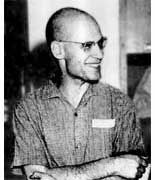The Oscar in the category The Best Rejected Research Proposal in Mathematics (ever) goes to … Alexander Grothendieck for his proposal Esquisse d’un Programme, Grothendieck\’s research program from 1983, written as part of his application for a position at the CNRS, the French equivalent of the NSF. An English translation is available. Here is one of the problems discussed : Give TWO non-trivial elements of$Gal(\overline{\mathbb{Q}}/\mathbb{Q}) $the _absolute_ Galois group of the algebraic closure of the rational numbers$\overline{\mathbb{Q}} $, that is the group of all$\mathbb{Q} $-automorphisms of$\overline{\mathbb{Q}} $. One element most of us can give (complex-conjugation) but to find any other element turns out to be an extremely difficult task. To get a handle on this problem, Grothendieck introduced his _’Dessins d’enfants’_ (Children’s drawings). Recall from last session the pictures of the left and right handed Monsieur Mathieu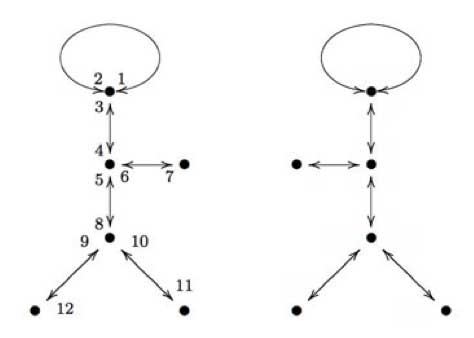The left hand side drawing was associated to a map$\mathbb{P}^1_{\mathbb{C}} \rightarrow \mathbb{P}^1_{\mathbb{C}} $which was defined over the field$\mathbb{Q} \sqrt{-11} $whereas the right side drawing was associated to the map given when one applies to all coefficients the unique non-trivial automorphism in the Galois group$Gal(\mathbb{Q}\sqrt{-11}/\mathbb{Q}) $(which is complex-conjugation). Hence, the Galois group$Gal(\mathbb{Q}\sqrt{-11}/\mathbb{Q}) $acts _faithfully_ on the drawings associated to maps$\mathbb{P}^1_{\mathbb{Q}\sqrt{-11}} \rightarrow
\mathbb{P}^1_{\mathbb{Q}\sqrt{-11}} $which are ramified only over the points${ 0,1,\infty } $. Grothendieck’s idea was to extend this to more general maps. Assume that a projective smooth curve (a Riemann surface) X is defined over the algebraic numbers$\overline{\mathbb{Q}} $and assume that there is a map$X
\rightarrow \mathbb{P}^1_{\mathbb{C}} $ramified only over the points${ 0,1,\infty } $, then we can repeat the procedure of last time and draw a picture on X consisting of d edges (where d is the degree of the map, that is the number of points lying over another point of$\mathbb{P}^1_{\mathbb{C}} $) between white resp. black points (the points of X lying over 1 (resp. over 0)). Call such a drawing a ‘dessin d\’enfant’ and look at the collection of ALL dessins d’enfants associated to ALL such maps where X runs over ALL curves defined over$\overline{\mathbb{Q}} $. On this set, there is an action of the absolute Galois group$Gal(\overline{\mathbb{Q}}/\mathbb{Q}) $and if this action would be faithful, then this would give us insight into this group. However, at that time even the existence of a map$X \rightarrow
\mathbb{P}^1 $ramified in the three points${ 0,1,\infty } $seemed troublesome to prove, as Grothendieck recalls in his proposal In more erudite terms, could it be true that every projective non-singular algebraic curve defined over a number field occurs as a possible ‚ modular curve‚ parametrising elliptic curves equipped with a suitable rigidification? Such a supposition seemed so crazy that I was almost embarrassed to submit it to the competent people in the domain. Deligne when I consulted him found it crazy indeed, but didn’t have any counterexample up his sleeve. Less than a year later, at the International Congress in Helsinki, the Soviet mathematician Bielyi announced exactly that result, with a proof of disconcerting simplicity which fit into two little pages of a letter of Deligne ‚ never, without a doubt, was such a deep and disconcerting result proved in so few lines! In the form in which Bielyi states it, his result essentially says that every algebraic curve defined over a number field can be obtained as a covering of the projective line ramified only over the points 0, 1 and infinity. This result seems to have remained more or less unobserved. Yet, it appears to me to have considerable importance. To me, its essential message is that there is a profound identity between the combinatorics of finite maps on the one hand, and the geometry of algebraic curves defined over number fields on the other. This deep result, together with the algebraic- geometric interpretation of maps, opens the door onto a new, unexplored world within reach of all, who pass by without seeing it. Belyi’s proof is indeed relatively easy (full details can be found in the paper Dessins d’enfants on the Riemann sphere by Leila Schneps). Roughly it goes as follows : as both X and the map are defined over$\overline{\mathbb{Q}} $the map is only ramified over (finitely many)$\overline{\mathbb{Q}} $-points. Let S be the finite set of all Galois-conjugates of these points and consider the polynomial$f_0(z_0) = \prod_{s \in S} (z_0 -s) \in
\mathbb{Q}[z_0] $Now, do a resultant trick. Consider the polynomial$f_1(z_1) = Res_{z_0}(\frac{d f_0}{d
z_0},f_0(z_0)-z_1) $then the roots of$f_1(z_1) $are exactly the finite critical values of$f_0 $,$f_1 $is again defined over$\mathbb{Q} $and has lower degree (in$z_1 $) than$f_0 $in$z_1 $. Continue this trick a finite number of times untill you have constructed a polynomial$f_n(z_n) \in \mathbb{Q}[z_n] $of degree zero. Composing the original map with the maps$f_j $in succession yields that all ramified points of this composition are$\mathbb{Q} $-points! Now, we only have to limit the number of these ramified$\mathbb{Q} $-points (let us call this set T) to three. Take any three elements of T, then there always exist integers$m,n
\in \mathbb{Z} $such that the three points go under a linear fractional transformation (a Moebius-function associated to a matrix in$PGL_2(\mathbb{Q}) $) to${ 0,\frac{m}{m+n},1 } $. Under the transformation$z \rightarrow \frac{(m+n)^{m+n}}{m^m
n^n}z^m(1-z)^n $the points 0 and 1 go to 0 and$\frac{m}{m+n} $goes to 1 whence the ramified points of the composition are one less in number than T. Continuing in this way we can get the set of ramified$\mathbb{Q} $-points of a composition at most having three elements and then a final Moebius transformation gets them to${ 0,1,\infty } $, done!As a tribute for this clever argument, maps$X \rightarrow \mathbb{P}^1 $ramified only in 0,1 and$\infty $are now called Belyi morphisms. Here is an example of a Belyi-morphism (and the corresponding dessin d’enfants) associated to one of the most famous higher genus curves around : the Klein quartic (if you haven’t done so yet, take your time to go through this marvelous pre-blog post by John Baez). One can define the Klein quartic as the plane projective curve K with defining equation in$\mathbb{P}^2_{\\mathbb{C}} $given by$X^3Y+Y^3Z+Z^3X = 0 $K has a large group of automorphism, namely the simple group of order 168$G = PSL_2(\mathbb{F}_7) =
SL_3(\mathbb{F}_2) $It is a classical fact (see for example the excellent paper by Noam Elkies The Klein quartic in number theory) that the quotient map$K \rightarrow K/G =
\mathbb{P}^1_{\mathbb{C}} $is ramified only in the points 0,1728 and$\infty $and the number of points of K lying over them are resp. 56, 84 and 24. Now, compose this map with the Moebius transormation taking${ 0,1728,\infty } \rightarrow { 0,1,\infty } $then the resulting map is a Belyi-map for the Klein quartic. A topological construction of the Klein quartic is fitting 24 heptagons together so that three meet in each vertex, see below for the gluing data-picture in the hyperbolic plane : the different heptagons are given a number but they appear several times telling how they must fit together)The resulting figure has exactly$\frac{7 \times 24}{2} =
84 $edges and the 84 points of K lying over 1 (the white points in the dessin) correspond to the midpoints of the edges. There are exactly$\frac{7 \times 24}{3}=56 $vertices corresponding to the 56 points lying over 0 (the black points in the dessin). Hence, the dessin d\’enfant associated to the Klein quartic is the figure traced out by the edges on K. Giving each of the 168 half-edges a different number one assigns to the white points a permutation of order two and to the three-valent black-points a permutation of order three, whence to the Belyi map of the Klein quartic corresponds a 168-dimensional permutation representation of$SL_2(\mathbb{Z}) $, which is not so surprising as the group of automorphisms is$PSL_2(\mathbb{F}_7) $and the permutation representation is just the regular representation of this group. Next time we will see how one can always associate to a curve defined over$\overline{\mathbb{Q}} $a permutation representation (via the Belyi map and its dessin) of one of the congruence subgroups$\Gamma(2) $or$\Gamma_0(2) $or of$SL_2(\mathbb{Z}) $itself. The Klein Four Group is an a capella group from the maths department of Northwestern. Below a link to one of their songs (grabbed from P.P. Cook’s Tangent Space ). Finite Simple Group (of order two) A Klein Four original by Matt Salomone The path of love is never smooth But mine’s continuous for you You’re the upper bound in the chains of my heart You’re my Axiom of Choice, you know it’s true But lately our relation’s not so well-defined And I just can’t function without you I’ll prove my proposition and I’m sure you’ll find We’re a finite simple group of order two I’m losing my identity I’m getting tensor every day And without loss of generality I will assume that you feel the same way Since every time I see you, you just quotient out The faithful image that I map into But when we’re one-to-one you’ll see what I’m about ‘Cause we’re a finite simple group of order two Our equivalence was stable, A principal love bundle sitting deep inside But then you drove a wedge between our two-forms Now everything is so complexified When we first met, we simply connected My heart was open but too dense Our system was already directed To have a finite limit, in some sense I’m living in the kernel of a rank-one map From my domain, its image looks so blue, ‘Cause all I see are zeroes, it’s a cruel trap But we’re a finite simple group of order two I’m not the smoothest operator in my class, But we’re a mirror pair, me and you, So let’s apply forgetful functors to the past And be a finite simple group, a finite simple group, Let’s be a finite simple group of order two (Oughter: “Why not three?”) I’ve proved my proposition now, as you can see, So let’s both be associative and free And by corollary, this shows you and I to be Purely inseparable. Q. E. D. Now that my non-geometry post is linked via the comments in this string-coffee-table post which in turn is available through a trackback from the Kontsevich-Soibelman paper it is perhaps useful to add a few links. The little I’ve learned from reading about Connes-style non-commutative geometry is this : if you have a situation where a discrete group is acting with a bad orbit-space (for example,$GL_2(\mathbb{Z})$acting on the whole complex-plane, rather than just the upper half plane) you can associate to this a$C^*$-algebra and study invariants of it and interprete them as topological information about this bad orbit space. An intruiging example is the one I mentioned and where the additional noncommutative points (coming from the orbits on the real axis) seem to contain a lot of modular information as clarified by work of Manin&Marcolli and Zagier. Probably the best introduction into Connes-style non-commutative geometry from this perspective are the Lecture on Arithmetic Noncommutative Geometry by Matilde Marcolli. To algebraists : this trick is very similar to looking at the skew-group algebra$\mathbb{C}[x_1,\ldots,x_n] * G$if you want to study the _orbifold_ for a finite group action on affine space. But as algebraist we have to stick to affine varieties and polynomials so we can only deal with the case of a finite group, analysts can be sloppier in their functions, so they can also do something when the group is infinite. By the way, the skew-group algebra idea is also why non-commutative algebraic geometry enters string-theory via the link with orbifolds. The easiest (and best understood) example is that of Kleinian singularities. The best introduction to this idea is via the Representations of quivers, preprojective algebras and deformations of quotient singularities notes by Bill Crawley-Boevey. Artin-style non-commutative geometry aka non-commutative projective geometry originated from the work of Artin-Tate-Van den Bergh (in the west) and Odeskii-Feigin (in the east) to understand Sklyanin algebras associated to elliptic curves and automorphisms via ‘geometric’ objects such as point- (and fat-point-) modules, line-modules and the like. An excellent survey paper on low dimensional non-commutative projective geometry is Non-commutative curves and surfaces by Toby Stafford and Michel Van den Bergh . The best introduction is the (also neverending…) book-project Non- commutative algebraic geometry by Paul Smith who maintains a noncommutative geometry and algebra resource page page (which is also available from the header). Non-geometry started with the seminal paper ‘Algebra extensions and nonsingularity’, J. Amer. Math. Soc. 8 (1995), 251-289 by Joachim Cuntz and Daniel Quillen but which is not available online. An online introduction is Noncommutative smooth spaces by Kontsevich and Rosenberg. Surely, different people have different motivations to study non-geometry. I assume Cuntz got interested because inductive limits of separable algebras are quasi-free (aka formally smooth aka qurves). Kontsevich and Soibelman want to study morphisms and deformations of$A_{\infty}$-categories as they explain in their recent paper . My own motivation to be interested in non-geometry is the hope that in the next decades one will discover new exciting connections between finite groups, algebraic curves and arithmetic groups (monstrous moonshine being the first, and still not entirely understood, instance of this). Part of the problem is that these three topics seem to be quite different, yet by taking group-algebras of finite or arithmetic groups and coordinate rings of affine smooth curves they all turn out to be quasi-free algebras, so perhaps non-geometry is the unifying theory behind these seemingly unrelated topics.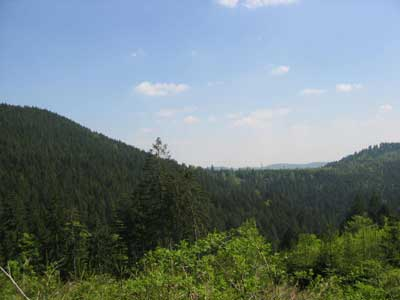If you go to Oberwolfach and the weather predictions are as good as last weeks , try to bring your mountain-bike along! Here is a nice 1hr30 to 2hrs tour : from the institute to Walke (height 300m), follow the road north to Rankach and at the Romanes Hof turn left to Hackerhof. Next, off-road along the Hacker lochweg until you hit the road Haaghutte-Mooshutte at an height of 855m (this should be doable in under one hour). A few metres further, you have a view at the highest mountains in the vicinity of the Institute : the Grosser Hundskopf (947m) and Kleiner Hundskopf (926m) as on the left. Then, descend along the Kirchhofweg over Moosbauerhof all the way down to the Dohlenbacherhof where you hit the main road which brings you back to the institute going SW. Please take a pump and repair material along, I had 2 flat tires in 4 days. If you happen to have a GPS, you can download the gpx-file. You can feed this to Tom Carden’s Google Map GPX Viewer and study it in more detail (I made one wrong turn in the descent and also briefly lost GPS reception in the forest near the top causing the top waypoint (the lower waypoint is the Institute)).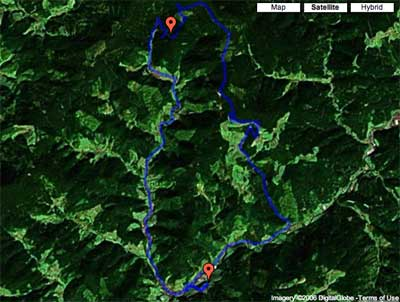If you were not present and still want to see some of the talks or if you are just curious in the outcome of Paul’s frantic typing on his PowerBook, he has put his (selection of talks)-notes online . Perhaps I’ll write down some of my own recollections of this meeting later. Klein’s quartic$X$is the smooth plane projective curve defined by$x^3y+y^3z+z^3x=0$and is one of the most remarkable mathematical objects around. For example, it is a Hurwitz curve meaning that the finite group of symmetries (when the genus is at least two this group can have at most$84(g-1)$elements) is as large as possible, which in the case of the quartic is$168$and the group itself is the unique simple group of that order,$G = PSL_2(\mathbb{F}_7)$also known as Klein\’s group. John Baez has written a [beautiful page](http://math.ucr.edu/home/baez/klein.html) on the Klein quartic and its symmetries. Another useful source of information is a paper by Noam Elkies [The Klein quartic in number theory](www.msri.org/publications/books/Book35/files/elkies.pd). The quotient map$X \rightarrow X/G \simeq \mathbb{P}^1$has three branch points of orders$2,3,7$in the points on$\mathbb{P}^1$with coordinates$1728,0,\infty$. These points correspond to the three non-free$G$-orbits consisting resp. of$84,56$and$24$points. Now, remove from$X$a couple of$G$-orbits to obtain an affine open subset$Y$such that$G$acts on its cordinate ring$\mathbb{C}[Y]$and form the Klein stack (or hereditary order)$\mathbb{C}[Y] \bigstar G$, the skew group algebra. In case the open subset$Y$contains all non-free orbits, the [one quiver](www.matrix.ua.ac.be/master/coursenotes/onequiver.pdf) of this qurve has the following shape$\xymatrix{\vtx{} \ar@/^/[dd] \\
\\ \vtx{} \ar@/^/[uu]} \xymatrix{& \vtx{} \ar[ddl] & \\
& & \\ \vtx{} \ar[rr] & & \vtx{} \ar[uul]} \xymatrix{& &
\vtx{} \ar[dll] & & \\ \vtx{} \ar[d] & & & & \vtx{} \ar[ull] \\ \vtx{}
\ar[dr] & & & & \vtx{} \ar[u] \\ & \vtx{} \ar[rr] & & \vtx{} \ar[ur]
&} $Here, the three components correspond to the three non-free orbits and the vertices correspond to the isoclasses of simple$\mathbb{C}[Y] \bigstar G$of dimension smaller than$168$. There are two such of dimension$84$, three of dimension$56$and seven of dimension$24$which I gave the non-imaginative names \’twins\’, \’trinity\’ and \’the dwarfs\’. As we want to spice up later this Klein stack to a larger group, we need to know the structure of these exceptional simples as$G$-representations. Surely, someone must have written a paper on the general problem of finding the$G$-structure of simples of skew-group algebras$A \bigstar G$, so if you know a reference please let me know. I used an old paper by Idun Reiten and Christine Riedtmann to do this case (which is easier as the stabilizer subgroups are cyclic and hence the induced representations of their one-dimensionals correspond to the exceptional simples). Here the story of an idea to construct new examples of non-commutative compact manifolds, the computational difficulties one runs into and, when they are solved, the white noise one gets. But, perhaps, someone else can spot a gem among all gibberish… [Qurves](http://www.neverendingbooks.org/toolkit/pdffile.php?pdf=/TheLibrary/papers/qaq.pdf) (aka quasi-free algebras, aka formally smooth algebras) are the \’affine\’ pieces of non-commutative manifolds. Basic examples of qurves are : semi-simple algebras (e.g. group algebras of finite groups), [path algebras of quivers](http://www.lns.cornell.edu/spr/2001-06/msg0033251.html) and coordinate rings of affine smooth curves. So, let us start with an affine smooth curve$X$and spice it up to get a very non-commutative qurve. First, we bring in finite groups. Let$G$be a finite group acting on$X$, then we can form the skew-group algebra$A = \mathbfk[X]
\bigstar G$. These are examples of prime Noetherian qurves (aka hereditary orders). A more pompous way to phrase this is that these are precisely the [one-dimensional smooth Deligne-Mumford stacks](http://www.math.lsa.umich.edu/~danielch/paper/stacks.pdf). As the 21-st century will turn out to be the time we discovered the importance of non-Noetherian algebras, let us make a jump into the wilderness and consider the amalgamated free algebra product$A =
(\mathbf k[X] \bigstar G) \ast_{\mathbf k G} \mathbfk H$where$G
\subset H$is an interesting extension of finite groups. Then,$A$is again a qurve on which$H$acts in a way compatible with the$G$-action on$X$and$A$is hugely non-commutative… A very basic example : let$\mathbb{Z}/2\mathbb{Z}$act on the affine line$\mathbfk[x]$by sending$x \mapsto -x$and consider a finite [simple group](http://mathworld.wolfram.com/SimpleGroup.html)$M$. As every simple group has an involution, we have an embedding$\mathbb{Z}/2\mathbb{Z} \subset M$and can construct the qurve$A=(\mathbfk[x] \bigstar \mathbb{Z}/2\mathbb{Z}) \ast_{\mathbfk
\mathbb{Z}/2\mathbb{Z}} \mathbfk M$on which the simple group$M$acts compatible with the involution on the affine line. To study the corresponding non-commutative manifold, that is the Abelian category$\mathbf{rep}~A$of all finite dimensional representations of$A$we have to compute the [one quiver to rule them all](http://www.matrix.ua.ac.be/master/coursenotes/onequiver.pdf) for$A$. Because$A$is a qurve, all its representation varieties$\mathbf{rep}_n~A$are smooth affine varieties, but they may have several connected components. The direct sum of representations turns the set of all these components into an Abelian semigroup and the vertices of the \’one quiver\’ correspond to the generators of this semigroup whereas the number of arrows between two such generators is given by the dimension of$Ext^1_A(S_i,S_j)$where$S_i,S_j$are simple$A$-representations lying in the respective components. All this may seem hard to compute but it can be reduced to the study of another quiver, the Zariski quiver associated to$A$which is a bipartite quiver with on the left the \’one quiver\’ for$\mathbfk[x] \bigstar
\mathbb{Z}/2\mathbb{Z}$which is just$\xymatrix{\vtx{}
\ar@/^/[rr] & & \vtx{} \ar@/^/[ll]} $(where the two vertices correspond to the two simples of$\mathbb{Z}/2\mathbb{Z}$) and on the right the \’one quiver\’ for$\mathbf k M$(which just consists of as many verticers as there are simple representations for$M$) and where the number of arrows from a left- to a right-vertex is the number of$\mathbb{Z}/2\mathbb{Z}$-morphisms between the respective simples. To make matters even more concrete, let us consider the easiest example when$M = A_5$the alternating group on$5$letters. The corresponding Zariski quiver then turns out to be$\xymatrix{& & \vtx{1} \\\
\vtx{}\ar[urr] \ar@{=>}[rr] \ar@3[drr] \ar[ddrr] \ar[dddrr] \ar@/^/[dd]
& & \vtx{4} \\\ & & \vtx{5} \\\ \vtx{} \ar@{=>}[uurr] \ar@{=>}[urr]
\ar@{=>}[rr] \ar@{=>}[drr] \ar@/^/[uu] & & \vtx{3} \\\ & &
\vtx{3}} $The Euler-form of this quiver can then be used to calculate the dimensions of the EXt-spaces giving the number of arrows in the \’one quiver\’ for$A$. To find the vertices, that is, the generators of the component semigroup we have to find the minimal integral solutions to the pair of equations saying that the number of simple$\mathbb{Z}/2\mathbb{Z}$components based on the left-vertices is equal to that one the right-vertices. In this case it is easy to see that there are as many generators as simple$M$representations. For$A_5$they correspond to the dimension vectors (for the Zariski quiver having the first two components on the left)$\begin{cases}
(1,2,0,0,0,0,1) \\ (1,2,0,0,0,1,0) \\ (3,2,0,0,1,0,0) \\
(2,2,0,1,0,0,0) \\ (1,0,1,0,0,0,0) \end{cases}$We now have all info to determine the \’one quiver\’ for$A$and one would expect a nice result. Instead one obtains a complete graph on all vertices with plenty of arrows. More precisely one obtains as the one quiver for$A_5\xymatrix{& & \vtx{} \ar@{=}[dll] \ar@{=}[dddl] \ar@{=}[dddr]
\ar@{=}[drr] & & \\\ \vtx{} \ar@(ul,dl)|{4} \ar@{=}[rrrr]|{6}
\ar@{=}[ddrrr]|{8} \ar@{=}[ddr]|{4} & & & & \vtx{} \ar@(ur,dr)|{8}
\ar@{=}[ddlll]|{6} \ar@{=}[ddl]|{10} \\\ & & & & & \\\ & \vtx{}
\ar@(dr,dl)|{4} \ar@{=}[rr]|{8} & & \vtx{} \ar@(dr,dl)|{11} & } $with the number of arrows (in each direction) indicated. Not very illuminating, I find. Still, as the one quiver is symmetric it follows that all quotient varieties$\mathbf{iss}_n~A$have a local Poisson structure. Clearly, the above method can be generalized easily and all examples I did compute so far have this \’nearly complete graph\’ feature. One might hope that if one would start with very special curves and groups, one might obtain something more interesting. Another time I\’ll tell what I got starting from Klein\’s quartic (on which the simple group$PSL_2(\mathbb{F}_7)$acts) when the situation was sexed-up to the sporadic simple Mathieu group$M_{24}$(of which$PSL_2(\mathbb{F}_7)\$ is a maximal subgroup).